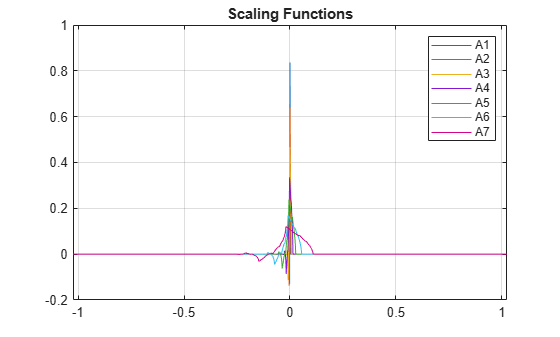# scalingfunctions

DWT filter bank time-domain scaling functions

## Syntax

``phi = scalingfunctions(fb)``
``[phi,t] = scalingfunctions(fb)``

## Description

````phi = scalingfunctions(fb)` returns the time-centered scaling functions for each level of the discrete wavelet transform (DWT) filter bank `fb`.```

example

````[phi,t] = scalingfunctions(fb)` returns the sampling instants, `t`.```

## Examples

collapse all

Create a seven-level DWT filter bank for a length 2048 signal, using the Daubechies `db2` wavelet and a sampling frequency of 1 kHz.

```wv = "db2"; len = 2048; Fs = 1e3; lev = 7; fb = dwtfilterbank('SignalLength',len,'Wavelet',wv,'Level',lev,'SamplingFrequency',Fs);```

Plot the scaling functions for each level of the filter bank.

```[phi,t] = scalingfunctions(fb); plot(t,phi') grid on xlim([-len/2*1e-3 len/2*1e-3]) title('Scaling Functions') legend('A1','A2','A3','A4','A5','A6','A7')```## Input Arguments

collapse all

Discrete wavelet transform (DWT) filter bank, specified as a `dwtfilterbank` object.

## Output Arguments

collapse all

Time-centered scaling functions of the filter bank `fb`, returned as a real-valued L-by-N matrix, where L is the filter bank `Level` and N is the `SignalLength`. The scaling functions are ordered in `phi` from the finest scale resolution to the coarsest scale resolution.

Sampling instants, returned as a real-valued vector `t` of length N, where N is the filter bank `SignalLength`. Sampling instants lie in the interval $\left[-\text{\hspace{0.17em}}½\text{\hspace{0.17em}}N\text{ }DT,\text{\hspace{0.17em}}\text{\hspace{0.17em}}½\text{\hspace{0.17em}}N\text{ }DT\right)$, where DT is the filter bank sampling period (reciprocal of the filter bank sampling frequency).

## Version History

Introduced in R2018a